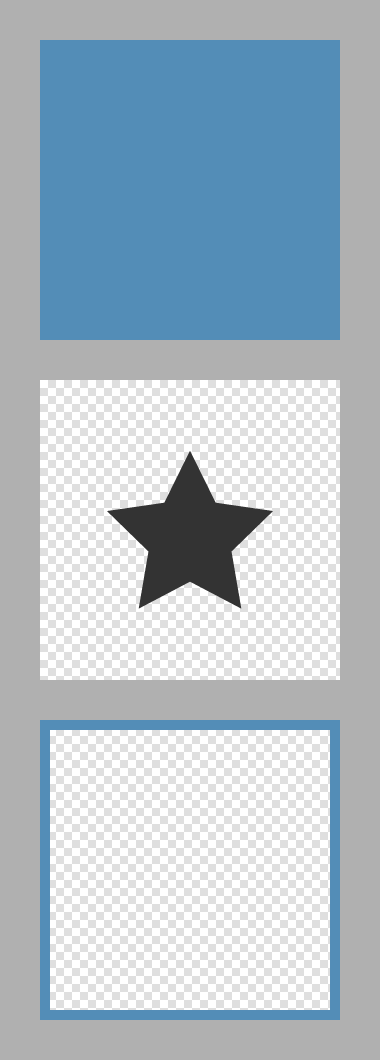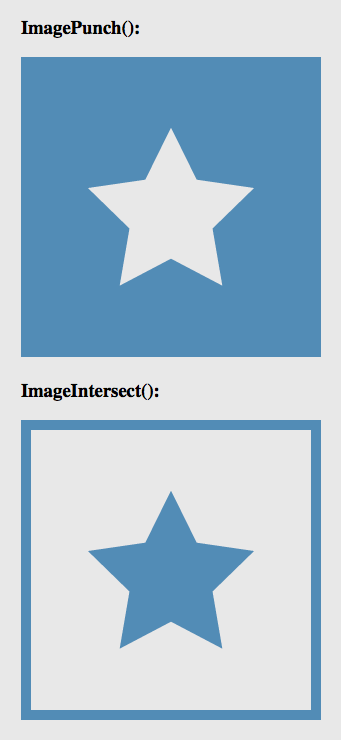On User Experience (UX) Design, JavaScript, ColdFusion, Node.js, Life, and Love.

# Using ImagePunch() And ImageIntersect() With ColdFusion

By on
Tags: ColdFusion

Earlier today, Evagoras Charalambous asked me about cropping a ColdFusion image on a diagonal. Cropping wasn't really what he wanted; but, since ColdFusion's list of native image functions doesn't have some advanced functionality, "cropping" is the terminology we're most comfortable with. What I believe Evagoras really wanted was a way to "punch" part of the image out. That is, to completely remove an irregular-shaped portion of the image, leaving the previous area transparent.

I didn't know how to do this offhand; but, after some Googling, I came across this tutorial by Ken on using the Java Graphics package to punch shapes out of a BufferedImage object. The Graphics package, in Java, provides for more advanced image manipulation techniques, including ways to define pixel-behaviors when creating a composite of two images.

After reviewing the Graphics2D and AlphaComposite JavaDocs, I decided to try using them to create two new ColdFusion image functions:

• imagePunch( img1, img2 ) - Punches the second image through the 1st image, leaving the intersecting pixels transparent.
• imageIntersect( img1, img2 ) - Punches the inverse of the second image through the 1st image, leaving and non-overlapping pixels transparent.

To start off, I created three test images: a rectangle, a star (with transparent background), and a border (with transparent background):I am going to try doing two different things: punching the star through the rectangle; and, intersecting the star with the rectangle. The border will subsequently be pasted over both resultant images, just as a sanity check.

In the following code, I define the two new ColdFusion image functions at the top and then demo them at the bottom. I have also created a utility function which duplicates a ColdFusion image object. Since image functions manipulate the underlying image, I need to duplicate the Rectangle so that I can use it in both methods.

NOTE: Neither the imageNew() nor the the duplicate() function properly duplicate images.

• <cffunction
• name="imagePunch"
• access="public"
• returntype="any"
• output="false"
• hint="I punch the second image through the first image.">
•
• <!--- Define arguments. --->
• <cfargument
• name="baseImage"
• type="any"
• required="true"
• hint="I am the ColdFssion Image that is being manipulated."
• />
•
• <cfargument
• name="punchImage"
• type="any"
• required="true"
• hint="I am the ColdFusion image that is being PUNCHED through the base image."
• />
•
• <cfargument
• name="punchX"
• type="numeric"
• required="false"
• default="0"
• hint="I am the X-coordinate of the top/left coordianate of the punch image."
• />
•
• <cfargument
• name="punchY"
• type="numeric"
• required="false"
• default="0"
• hint="I am the Y-coordinate of the top/left coordianate of the punch image."
• />
•
• <!---
• In order to manipulate the base image in more complex ways,
• we're going to create a Graphics instance as a proxy to the
• underlying base image data.
• --->
• <cfset local.graphics = imageGetBufferedImage( baseImage )
• .createGraphics()
• />
•
• <!---
• Set the compisite rules for the graphics engine to replace
• all pixles in the base that correspond to pixels in the Punch
• image with an alpha transparency.
•
• We are punching "OUT" any pixels in the base image that have
• overlapping pixels in the punch image.
• --->
• <cfset local.graphics.setComposite(
• createObject( "java", "java.awt.AlphaComposite" ).DstOut
• ) />
•
• <!---
• Draw the punch image over the base image - let the composite
• setting render the punch.
• --->
• <cfset local.graphics.drawImage(
• imageGetBufferedImage( punchImage ),
• javaCast( "int", punchX ),
• javaCast( "int", punchY ),
• javaCast( "null", "" )
• ) />
•
• <!---
• Return the mutated base image (since the image is passed by-
• reference, there's no real need to return it - the original
• image has been mutated).
• --->
• <cfreturn baseImage />
•
• </cffunction>
•
•
• <cffunction
• name="imageIntersect"
• access="public"
• returntype="any"
• output="false"
• hint="I remove any part of the base image that does not overlap with the intersect image.">
•
• <!--- Define arguments. --->
• <cfargument
• name="baseImage"
• type="any"
• required="true"
• hint="I am the ColdFssion Image that is being manipulated."
• />
•
• <cfargument
• name="intersectImage"
• type="any"
• required="true"
• hint="I am the ColdFusion image that is defining the portion of the base image to keep."
• />
•
• <cfargument
• name="intersectX"
• type="numeric"
• required="false"
• default="0"
• hint="I am the X-coordinate of the top/left coordianate of the intersect image."
• />
•
• <cfargument
• name="intersectY"
• type="numeric"
• required="false"
• default="0"
• hint="I am the Y-coordinate of the top/left coordianate of the intersect image."
• />
•
• <!---
• In order to manipulate the base image in more complex ways,
• we're going to create a Graphics instance as a proxy to the
• underlying base image data.
• --->
• <cfset local.graphics = imageGetBufferedImage( baseImage )
• .createGraphics()
• />
•
• <!---
• Set the compisite rules for the graphics engine to replace
• all pixles in the base image that do NOT correspond to pixels
• in the intersect image with an alpha transparency.
•
• We are punching "OUT" any pixels in the base image that do
• not overlap with pixels in the intersect image.
• --->
• <cfset local.graphics.setComposite(
• createObject( "java", "java.awt.AlphaComposite" ).DstIn
• ) />
•
• <!---
• Draw the intersect image over the base image - let the
• composite setting render the intersection.
• --->
• <cfset local.graphics.drawImage(
• imageGetBufferedImage( intersectImage ),
• javaCast( "int", intersectX ),
• javaCast( "int", intersectY ),
• javaCast( "null", "" )
• ) />
•
• <!---
• Return the mutated base image (since the image is passed by-
• reference, there's no real need to return it - the original
• image has been mutated).
• --->
• <cfreturn baseImage />
•
• </cffunction>
•
•
• <cffunction
• name="imageDuplicate"
• access="public"
• returntype="any"
• output="false"
• hint="I duplicate the given image, cicumventing ColdFusion's attempt to optimize image duplication.">
•
• <!--- Define arguments. --->
• <cfargument
• name="image"
• type="any"
• required="true"
• hint="I am the ColdFusion image being duplicated."
• />
•
• <!--- Return the full copy of the given image. --->
• <cfreturn imageCopy(
• image,
• 0,
• 0,
• imageGetWidth( image ),
• imageGetHeight( image )
• ) />
•
• </cffunction>
•
•
• <!--- ----------------------------------------------------- --->
• <!--- ----------------------------------------------------- --->
• <!--- ----------------------------------------------------- --->
• <!--- ----------------------------------------------------- --->
•
•
• <!DOCTYPE html>
• <html>
• <title>ImagePunch() And ImageIntersect() With ColdFusion</title>
•
• <style type="text/css">
•
• /*
• * Set a background color on the page so that we can see
• * where the punching and the intersection have left alpha-
• * transparencies in our base images.
• */
• body {
• background-color: #E8E8E8 ;
• }
•
• </style>
• <body>
•
• <!--- Load in our three images. --->
• <cfset rectangle = imageNew( "./rectangle.png" ) />
• <cfset star = imageNew( "./star.png" ) />
• <cfset border = imageNew( "./border.png" ) />
•
• <!---
• Now, let's punch the star through the rectangle. When we do
• this, we want to duplicate the rectangle since the punch will
• alter the underlying image and we want to use the image more
• than once.
• --->
• <cfset punchedRectangle = imagePunch(
• imageDuplicate( rectangle ),
• star
• ) />
•
• <!--- Let's also try to intersect the star and the rectangle. --->
• <cfset intersectedRectangle = imageIntersect(
• imageDuplicate( rectangle ),
• star
• ) />
•
• <!--- Add the border to both images. --->
• <cfset imagePaste( punchedRectangle, border, 0, 0 ) />
• <cfset imagePaste( intersectedRectangle, border, 0, 0 ) />
•
•
• <!--- Draw the images to the browser. --->
•
•
• <h3>
• ImagePunch():
• </h3>
•
• <cfimage
• source="#punchedRectangle#"
• action="writeToBrowser"
• />
•
• <h3>
• ImageIntersect():
• </h3>
•
• <cfimage
• source="#intersectedRectangle#"
• action="writeToBrowser"
• />
•
• </body>
• </html>

As you can see, we are using the underlying Graphics2D and AlphaComposite classes to define how overlapping pixels behave when the two images are merged. And when we run the above code, the following images get written the browser. Notice that I have made the background color of the page light-gray so that we can see the transparencies in the resultant PNGs:As you can see, imagePunch() punched the star through the rectangle; and, imageIntersect() punched the inverse of the star through the rectangle. Pretty cool stuff! Being able to punch random shapes through an object really opens the door for very cool, on-the-fly image generation.Obviously you are going to put this in imageUtils, right??? :)@Ray,

Definitely - this just had to be done quickly - was for a client project :DWow, that was blazingly fast! Forget Google; you are even faster with questions. I need to go through this now in detail. Thanks for taking the time to figure this out!